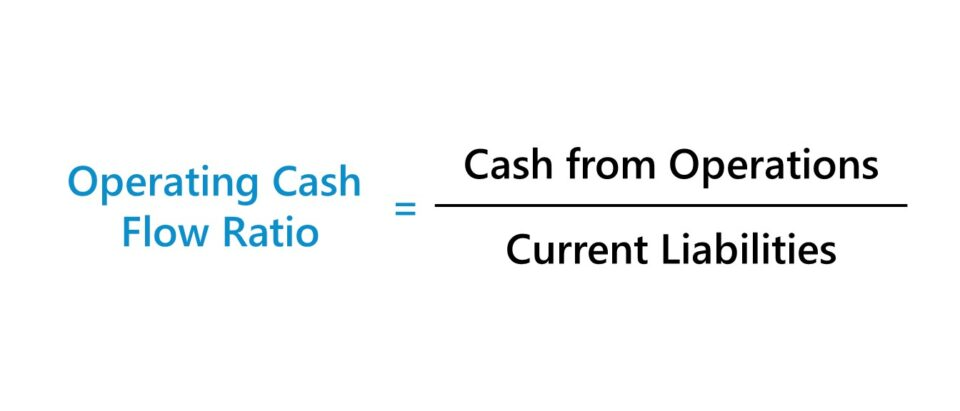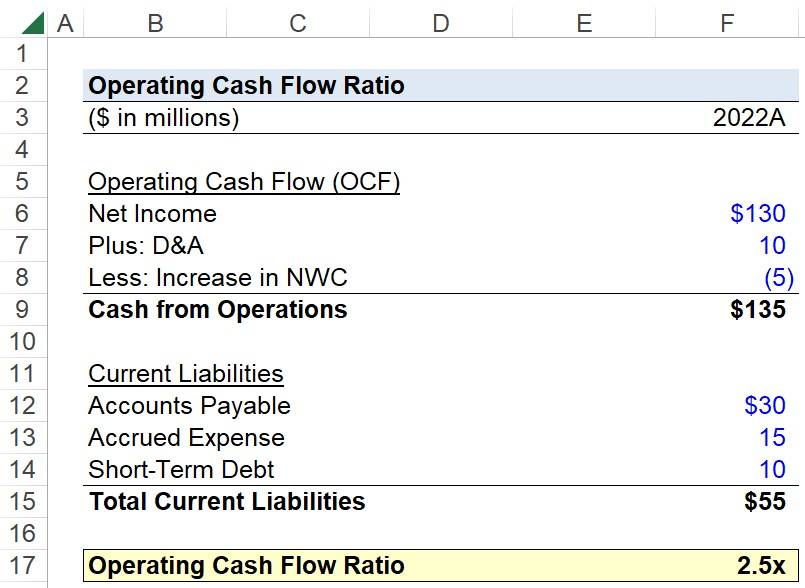Welcome to Wall Street Prep! Use code at checkout for 15% off.# Operating Cash Flow Ratio

Guide to Understanding the Operating Cash Flow Ratio## How to Calculate Operating Cash Flow Ratio?

The operating cash flow ratio (OCF) evaluates the near-term liquidity risk of a company by comparing its cash flows from operations, i.e. core business activities, and current liabilities.

The two metrics that the OCF ratio compares can be found on the cash flow statement (CFS) and balance sheet (B/S).

1. Operating Cash Flow (OCF): The operating cash flow, or cash flow from operations (CFO), is equal to a company’s accrual-based net income adjusted for non-cash items like depreciation and amortization (D&A) and changes in net working capital (NWC).
2. Current Liabilities: The current liabilities of a company are the unmet obligations (i.e. future cash outflows) that come due within the next twelve months, i.e. one year. Some of the more common examples of current liabilities that appear on the balance sheet are accounts payable (A/P), accrued expenses, and short-term debt (or the short-term portion of long-term debt).

The operating cash flow ratio can be interpreted as, “How much operating cash flows does the company’s core business activities produce for each dollar of current liabilities?”

As a result, a higher OCF ratio is preferred from a risk standpoint, because that suggests the company brings in enough operating cash flows to fulfill its short-term obligations.

The reliance on the OCF ratio as a measure of liquidity might not be as prevalent as other metrics, such as the current ratio, quick ratio, and interest coverage ratio.

However, the OCF ratio is still useful to evaluate a company’s short-term liquidity, because the cash flow metric is adjusted for accruals.

## Operating Cash Flow Ratio Formula (OCF)

The formula for calculating the operating cash flow ratio is as follows.

Operating Cash Flow Ratio = Cash from Operations ÷ Current Liabilities

## Operating Cash Flow Ratio Calculator

We’ll now move on to a modeling exercise, which you can access by filling out the form below.Submitting...

## Operating Cash Flow Ratio Calculation Example (OCF)

Suppose you’re tasked with calculating the operating cash flow ratio of a company, given the following assumptions.

Cash Flow Statement (CFS)

• Net Income = \$130 million
• D&A = \$10 million
• Increase in NWC = (\$5 million)

Current Liabilities

• Accounts Payable = \$30 million
• Accrued Expense = \$15 million
• Short-Term Debt = \$10 million

The company’s cash from operations is \$135 million, while its current liabilities are \$55 million.

• Cash from Operations = \$130 million + \$10 million – \$5 million = \$135 million
• Total Current Liabilities = \$30 million + \$15 million + \$10 million = \$55 million

After entering our two metrics into the formula from earlier, the calculated operating cash flow ratio is 2.5x, meaning the company’s operating cash flow can cover its current liability obligations two and a half times.Step-by-Step Online Course

### Everything You Need To Master Financial Modeling

Enroll in The Premium Package: Learn Financial Statement Modeling, DCF, M&A, LBO and Comps. The same training program used at top investment banks.